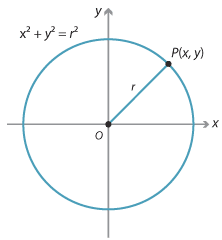### Equation of a circle

#### Circles with centre the origin

Consider a circle in the coordinate plane with centre the origin and radius r.Detailed description

If P(x, y) is a point on the circle, its distance from the origin is r and, by Pythagoras' theorem, $$x^2 + y^2 = r^2$$.
Conversely, if a point P(x, y) in the plane satisfies the equation $$x^2 + y^2 = r^2$$, its distance from O(0, 0) is r, so it lies on a circle with centre the origin and radius r.

#### Summary

The circle with centre O(0, 0) and radius r has equation $$x^2 + y^2 = r^2$$.